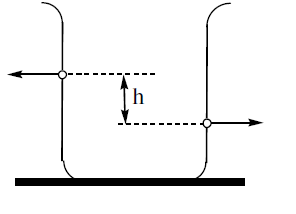Questions

# There are two identical small holes of area of cross section a on the opposite sides of a tank containing a liquid of density $\rho$ . The difference in height between the holes is h. The tank is resting on a smooth horizontal surface. The horizontal force which will have to be applied on the tank to kept it in equilibrium isModerate
A

B

$\frac{2\mathrm{gh}}{\rho \mathrm{a}}$

C

D

$\frac{\rho gh}{a}$